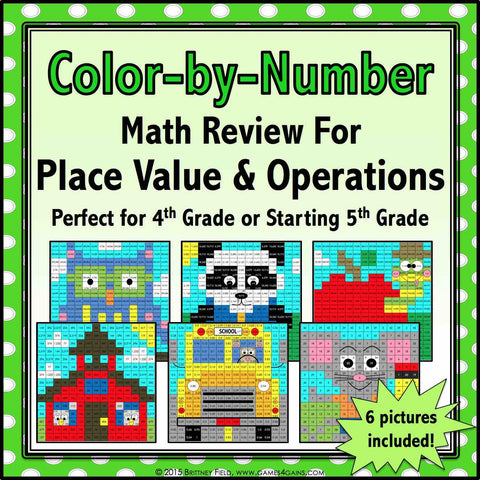## Back to School Color-by-Number - 4th/5th Grade

• \$480

This back to school set comes with 6 "School Days" math color-by-number activities for reviewing and practicing place value and operations standards from 4th grade. This set is perfect to use as review with incoming 5th graders or as practice with 4th graders who have already learned these skills.

Included with this "School Days" color-by-number set:
Instructions
6 color by number worksheets
6 student problem worksheets

Here are the Common Core standards covered by the color-by-number activities:

CCSS.MATH.CONTENT.4.NBT.A.2
Read and write multi-digit whole numbers using base-ten numerals, number names, and expanded form.

CCSS.MATH.CONTENT.4.NBT.A.3
Use place value understanding to round multi-digit whole numbers to any place.

CCSS.MATH.CONTENT.4.NBT.B.5
Multiply a whole number of up to four digits by a one-digit whole number.

CCSS.MATH.CONTENT.4.NBT.B.6
Find whole-number quotients and remainders with up to four-digit dividends and one-digit divisors.

CCSS.MATH.CONTENT.4.NF.C.6
Use decimal notation for fractions with denominators 10 or 100.

CCSS.MATH.CONTENT.4.OA.B.4
Find all factor pairs for a whole number in the range 1-100.